Polynomials MathBitsNotebook.com Terms of Use   Contact Person: Donna Roberts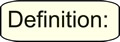A polynomial is a finite sum of terms in which all variables have whole number exponents and no variable appears in a denominator.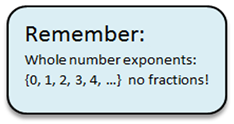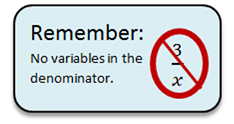The leading term in a polynomial is the term of highest degree.
The constant term in a polynomial is the term without a variable.

 Polynomials: YES or NO 3x½ NO - exponent is not a whole number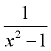NO - the variable is in the denominator -3x2 YES - satisfies the polynomial definition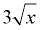NO - the square root of x can be written with a fractional exponent of x. 2x-3 NO - exponent is not a whole number. Negative exponent places x3 in denominator.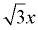YES - the exponent of x is 1 which is OK. The coefficient being radical 3 is not a problem.

 Classification by Terms monomial one term: 12, 4x, x2, -5xy binomial two terms: 2x - 1, x2 - 4 trinomial three terms: x2 + 2x + 1 polynomial - one or more terms: polynomial means "many", but it can also be one term. The ending of these words "nomial" is Greek for "part". Classification by Degree Linear - degree of 1 or 0:    3x + 1  or  12 Quadratic - degree of 2:   2x2 - x + 7 Cubic - degree of 3:   3x3 + 4x2 + 3x + 5The degree of a term with whole number exponents is the sum of the exponents of the variables, if there are variables. Non-zero constants have degree 0, and the term zero has no degree.  Example: 6x2 has a degree of 2;   4x2y3 has a degree of 5 (the sum of 2 and 3).The degree of a polynomial is the highest degree of its terms. Example: 3x2 + 4x + 1 has a degree of 2;  x3 - x2 + 5x - 2 has a degree of 3
 The standard form of a polynomial is when all like terms are combined and the degrees are arranged in descending order. Polynomial: 2x + 3x5 + 4x3 - 8 Standard form: 3x5 + 4x3 + 2x - 8 3x5 + 4x3 + 2x1 - 8x0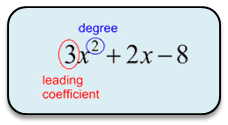Will we ever really use the degree? Knowing the "degree" of a polynomial function will let you determine the most number of solutions the function may have, and the most number of times the function will cross the x-axis. FYI: The standard form of a polynomial is formally written as anxn + an - 1xn - 1 + ... + a2x2 + a1x + a0, where n is a non-negative integer, and a0, a1, a2, ..., an are real number constant coefficients with an ≠ 0.Polynomials and Closure:
Polynomials form a system similar to the system of integers, in that polynomials are closed under the operations of addition, subtraction, and multiplication.

 CLOSURE: Polynomials will be closed under an operation if the operation produces another polynomial.

Closure under Addition: (2x2 + 3x + 4) + (x2 - 5x - 3) = 3x2 - 2x + 1
When adding polynomials, the variables and their exponents do not change. Only their coefficients will possibly change. This guarantees that the sum has variables and exponents which are already classified as belonging to polynomials. Polynomials are closed under addition.

Closure under Subtraction: (2x2 + 3x + 4) - (x2 - 5x - 3) = x2 + 8x + 7
When subtracting polynomials, the variables and their exponents do not change. Only their coefficients will possibly change. This guarantees that the difference has variables and exponents which are already classified as belonging to polynomials. Polynomials are closed under subtraction.

Closure under Multiplication: (x + 1)(x2 + 4x + 3) = x3 + 5x2 + 7x + 3
When multiplying polynomials, the variables' exponents are added, according to the rules of exponents. Remember that the exponents in polynomials are whole numbers. The whole numbers are closed under addition, which guarantees that the new exponents will be whole numbers. Consequently, polynomials are closed under multiplication.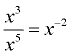When dividing variables with exponents, there is the possibility of creating a negative exponent. Negative exponents are not allowed in polynomials.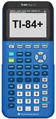For help with polynomials on your calculator, click add, mult. and/or square, divide.